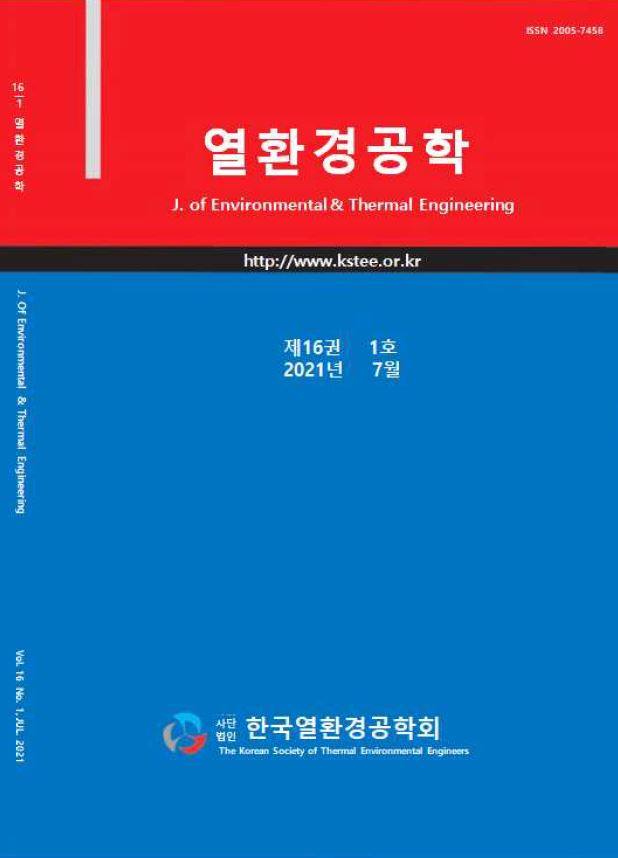상세검색
최근 검색어 전체 삭제
다국어입력
즐겨찾기0KCI등재후보 학술저널

# Calculation of Thermochemical Kinetic Parameters of Several Types of Biomass Using Friedman Isoconversional Method

• 등재여부 : KCI등재후보
• 2021.07
• 11 - 21 (11 pages)
• 12

In the previous study, the activation energy of waste coffee grounds using the Kissinger model was 42.57 kJ/mol. In the case of the Friedman model, the activation energy value ranged from 44.01 to 350.20 kJ/mol at each conversion rate, while the average activation energy value recorded was 127.73 kJ/mol. In the case of the Kissinger model, the calculation method was simple and the activation energy value could be obtained in a short time, but the activation energy value was found to be low in the case of different pyrolysis characteristics as influenced by temperature. Since the Friedman model was able to obtain the activation energy value at each conversion rate change, it was suitable for biomass kinetic analysis. In the case of seven types of biomass from Philippines in Southeast Asia, the Friedman model used after several stages of data processing was applied to calculate the effective activation energy value from TGA data. In this study, processing data of biomass thermogravimetric analysis can help the estimation of kinetic parameters by reducing the error caused by the differential values of the reaction conversion rate at each operating temperature.

1. Introduction

2. Materials and Method

3. Results and discussion

4. Conclusion